# DAV Class 6 Maths Chapter 1 Worksheet 3 Solutions

The DAV Class 6 Maths Book Solutions Pdf and DAV Class 6 Maths Chapter 1 Worksheet 3 Solutions of Natural Numbers and Whole Numbers offer comprehensive answers to textbook questions.

## DAV Class 6 Maths Ch 1 WS 3 Solutions

Question 1.
Fill in the blanks to make the following statements true.

(a) 1794 + 624 = 624 + _____
Solution:
1794

(b) (287 + 163) + 800 = 287 + (_____ + 800)
Solution:
163

(c) 432 + _____ = 111 + 432
Solution:
111

(d) 97 + 561 = _____ + 97
Solution:
561

(e) (200 + 1020) + 3303 = _____ + (200 + _____)
Solution:
3303, 1020

(f) 0 + 268 = _____
Solution:
268

(g) 469 – 0 = _____
Solution:
469

(h) 1238 – _____ = 1238
Solution:
0

(i) 29487 + _____ = 29487
Solution:
0Question 2.
Replace (*) with the appropriate digit.

(a)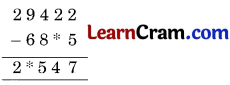Solution:(b)Solution: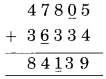(c)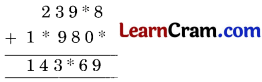Solution: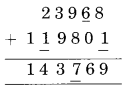(d)Solution: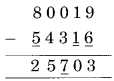Question 3.
Add 718662 to 360895. Now add 360895 to 718662. Are the two results same?
Solution:The both results are the same.

Question 4.
Add the following numbers by re-arranging them. (Use property here)

(a) 786 + 342 + 214
(b) 479 + 2000 + 21
(c) 225 + 725 + 275 + 275
(d) 67 + 1376 + 624 + 933
(e) 637 + 908 + 363
(f) 2062 + 547 + 938 + 353
Solution:
(a) 786 + 342 + 214
= 786 + (342 + 214)
= 786 + 556
= 1342

(b) 479 + 2000 + 21
= 2000 + (479 + 21)
= 2000 + 500
= 2500

(c) 225 + 725 + 275 + 275
= (225 + 725) + (275 + 275)
= 950 + 550
= 1500

(d) 67 + 1376 + 624 + 933
= (67 + 933) + (1376 + 624)
= 1000 + 2000
= 3000

(e) 637 + 908 + 363
= (637 + 363) + 908
= 1000 + 908
= 1908

(f) 2062 + 547 + 938 + 353
= (2062 + 938) + (547 + 353)
= 3000 + 900
= 3900Question 5.

(a) 29435 – 17005
Solution: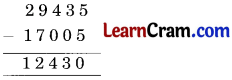Check:∴ 29435 – 17005 = 12430
29435 = 12430 + 17005
29435 = 29435
Hence verified.

(b) 100000 – 62581
Solution: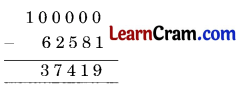Check:∴ 100000 – 62581 = 37419
100000 = 37419 + 62581
100000 = 100000
Hence verified.

(c) 75691 – 45512
Solution:Check: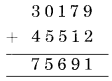∴ 75691 – 45512 = 30179
75691 = 30179 + 45512 75691 = 75691
Hence verified.

(d) 77426 – 71236
Solution: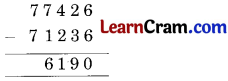Check:∴ 77426 – 71236 = 6190
77426 = 71236 + 6190
77426 = 77426
Hence verified.Question 6.
In a school, the number of students is 5637. If 142 students took admission during that year, find the total number of students in the school.
Solution:
Number of students = 5637
Number of admitted students = 142
∴ Total number of students = 5637 + 142 = 5779.

Question 7.
The price of a car is ₹ 3,76,866. If it is increased by ₹ 42049 find the new price of the car.
Solution:
Price of car = ₹ 3,76,866
Increased price of the Car = ₹ 42049.
∴ Total price of the Car = ₹ 3,76,866 + ₹ 42,049 = 376866Hence, the total price of the Car = ₹ 4,18,915.Question 8.
A club organises a trip to Disney World. The cost of the whole package is ₹ 1,83,420. The club gives a discount of ₹ 47,632. What is the cost of the package after the discount?
Solution:
Cost of the package = ₹ 1,83,420
Discount = ₹ 47632 .
∴ Net cost of the package = ₹ 1,83,420 – ₹ 47632Hence, the net value of the package = ₹ 1,35,788.

Question 9.
Rahul deposited ₹ 57,630 in the bank. After a week, he withdrew ₹ 19,211. What is the current balance in Rahul’s account?
Solution:
Money deposited = ₹ 57,630
Withdrawal = ₹ 19,211
∴ Balance in Rahul’s account = ₹ 57,630 – ₹ 19,211Hence, the balance in account = ₹ 38,419.

Question 10.
A garment factory produces 33,000 trousers every year. Out of these, 12309 are for men and 9538 are for women. Find the number of trousers produced for children.
Solution:
Trousers for children = 33000 – (12309 + 9538)
= 33000 – 21847
= 11153Hence, the number of trousers produced for children = 11153.### DAV Class 6 Maths Chapter 1 Worksheet 3 Notes

Operations on Whole Numbers:

(i) a + b = b + a
(ii) a + (b + c) = (a + b) + c

2. Subtraction:
(i) a – b = Whole number if a > b
(ii) b – a = Not a whole number if a > b

3. Multiplication:
(i) a × b = b × a
(ii) a × (b × c) = (a × b) × c = (a × c) × b
(iii) a × 0 = 0 × a = 0
(iv) a × 1 = 1 × a = a

4. Division:
If a and b are any two whole numbers, then
(i) a ÷ b = may or may not be a whole number
(ii) 0 ÷ a = 0
(iii) a ÷ 0 = meaningless
(iv) a ÷ 1 = aDistributive property:

a × (b + c) = a × b + a × c

Example 1:
Add 38, 65 and 94 in three different ways.
Solution:
(i) 38 + 65 + 94 = 38 + (65 + 94)
= 38 + 159
= 197

(ii) 38 + 65 + 94 = (38 + 65) + 94
= 103 + 94
= 197

(iii) 38 + 65 + 94 = 65 + (38 + 94)
= 65 + 132
= 197

Example 2:
Subtract 34 from 97
Solution:
97 – 34 = 63 or
97 = 34 + 63

Example 3:
Multiply 8, 9
Solution:
Using distributive property
8 × 9 = 8 × (10 – 1)
= 8 × 10 – 8 × 1
= 80 – 8
= 72Example 4:
283 × 98
Solution:
283 × 98 = 283 × (100 – 2)
= 28300 – 283 × 2
= 28300 – 566
= 27734

Example 5:
Solve: 38 × 55 + 42 × 55
Solution:
38 × 55 + 42 × 55 55 × (38 +42)
= 55 × 80
= 4400

Example 6:
Divide 2853 by 62 and check the answer.
Solution:Check:
Dividend = Quotient × Divisor + Remainder
2853 = 46 × 62 + 1
2853 = 2852 + 1
∴ 2853 = 2853